# Introduction to Regression Using Gaussian Processes

## Introduction

When trying to describe data using a function you often know something about the process generating the data a priori. When you do not completely understand why the data looks like it does but want to try to describe it any way you can start trying different things ad hoc.

A couple of years ago I began working with statistical methods to describe data. For points of a (continuous and smooth) function in a relevant neighborhood, it is not unreasonable to assume that values of these points do not differ much. Points close to each other experience a high level of correlation while points farther away are less correlated (but not necessarily if we experience periodic behavior). Given a set of points we want to evaluate in a function, we could introduce a multidimensional joint statistical distribution which describes how each point is related to all other points. The Gaussian Process is one way of doing this by assuming that all points are related to each other based on a joint Gaussian distribution.

Gaussian Processes are usually mentioned as a “parameterless” regression method. Parameterless is valid to some extent, but in practice, there are a set of so called hyper parameters which need to be tuned. To obtain a numerical value of the amount of covariance between different points we can use a kernel function. The squared exponential is a common function to start with:$kleft(x, x'right) = -frac{left||x - x'right||^2_2}{2 ell^2}$. The parameter$ell$ controls how smooth the functions described by the joint Gaussian distribution are and can be seen as a length-scale.

## Drawing Random Functions from the Prior Distribution

The first thing we can do is to draw some realizations from the distribution. We start by defining a set of points evenly distributed between -1 and 1 called$mathbf{x}_*$. For these points, we want to obtain random function values distributed according to$f(mathbf{x}) sim mathcal{GP}left(m(mathbf{x}), K(mathbf{x}, mathbf{x'})right)$, where$m(mathbf{x})$ is a mean value function and$k(mathbf{x}, mathbf{x'})$ the correlation matrix.

We need to calculate how each point in the the set$mathbf{x}_*$ is related to all other points given the kernel function$K(mathbf{x}_*, mathbf{x}_*) = left( begin{array}{cccc} k(x_1, x_1) & k(x_1, x_2) & cdots & k(x_1, x_n) \ k(x_2, x_1) & k(x_2, x_2) & cdots & k(x_2, x_n) \ vdots & vdots & ddots & vdots \ k(x_n, x_1) & k(x_n, x_2) & cdots & k(x_n, x_n) end{array} right)$,

followed by generating the functions$f_* sim mathcal{N}left(m, Kright)$ by calculating$fleft(x_*right) = m + Lu$ where$u sim mathcal{N}left(0, Iright)$ and$L$ is the lower triangular Cholesky factorization$K = LL^T$ (sometimes called the square root of matrices). We are setting$m = 0$ to have the distribution for the functions centered around zero.

Generating three random such functions using$ell = 0.3$ gives the following figure (for our random number generator) where the gray area shows +/- 1.96 standard deviations.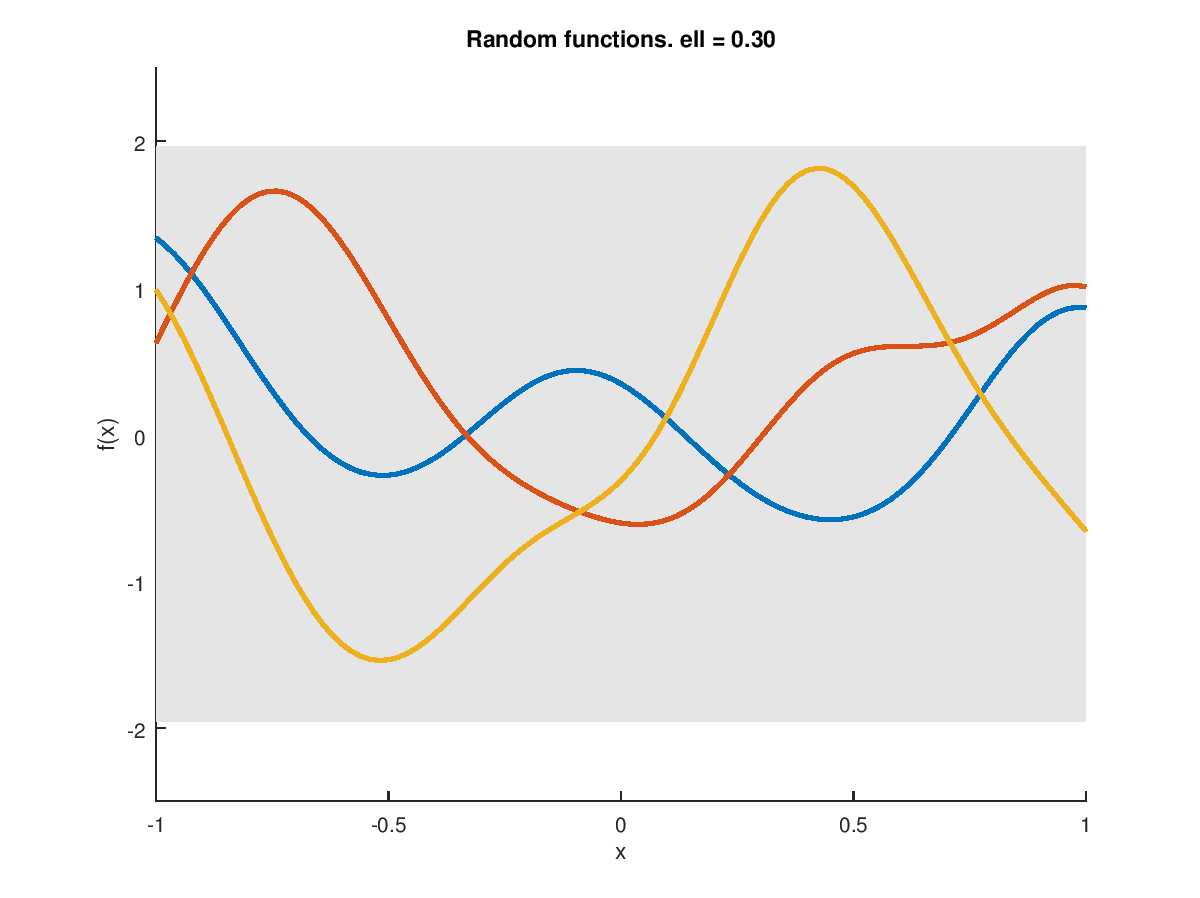The generated functions are continuous and smooth, and their curvature is similar to each other. Interesting things start to happen when we throw prior knowledge on the calculations.

## Drawing Random Functions from the Posterior Distribution

Assume that we have measured a sample at$x = 0$ which is$y(0) = 1$. We would like to draw new random functions from the distribution given this measurement. The posterior functions are distributed according to$mathbf{f_*} | X_*,X,mathbf{y} sim mathcal{N} left( K(X_*,X)K(X,X)^{-1}mathbf{y}, K(X_*,X_*)-K(X_*,X)K(X,X)^{-1}K(X,X_*) right)$

where the symbols subscripted with * represent points we want to evaluate, and those without * are observations.

The same procedure is used as in the previous case resulting in the following figure. Our sample is shown as a black filled circle. All generated functions are crossing the point, and the gray area becomes small around the point telling us that it is certain that the function must contain the point.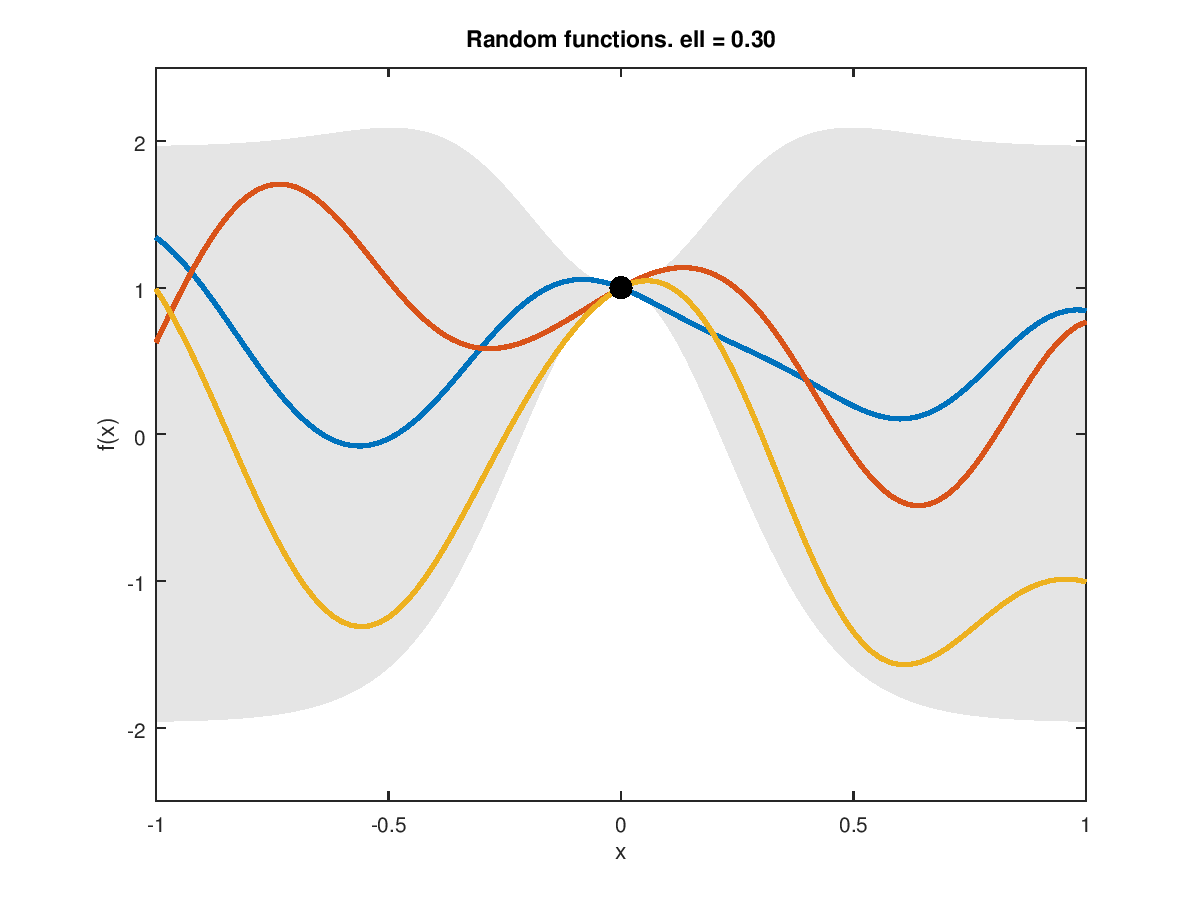Adding two more points restricts the possible outcome even more. The functions must pass through the three points since they are regarded as “truth.” Due to the limited curvature, the functions must focus on getting to the next point in-between.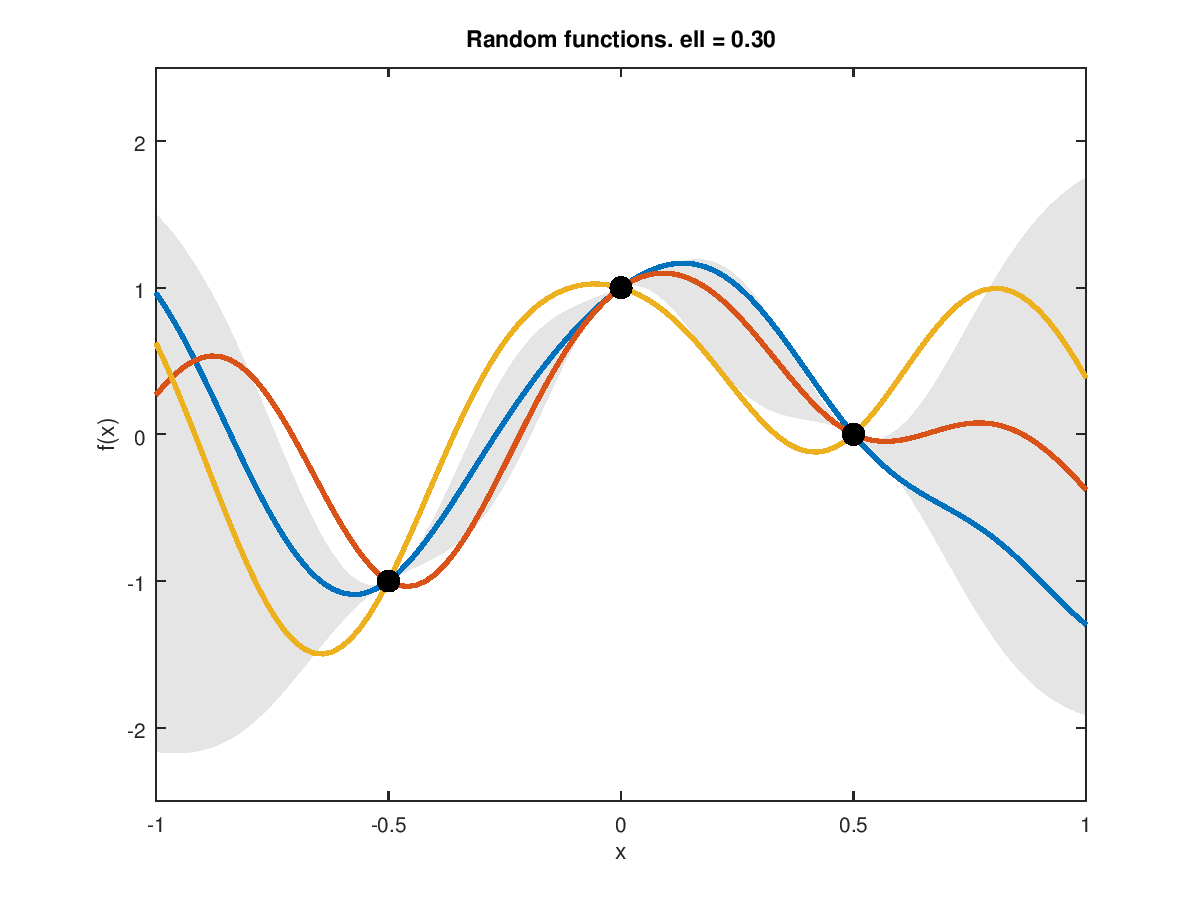Fine, generating random functions is nice, but what else can we do? Since the functions are drawn from a joint Gaussian distribution, we can calculate the expected value of the distribution.

## Regression Using the Expected Function

The expected function for our prior distribution example is just zero. For the case with one measurement point, we get a line which approaches zero at the edges and passes through the point.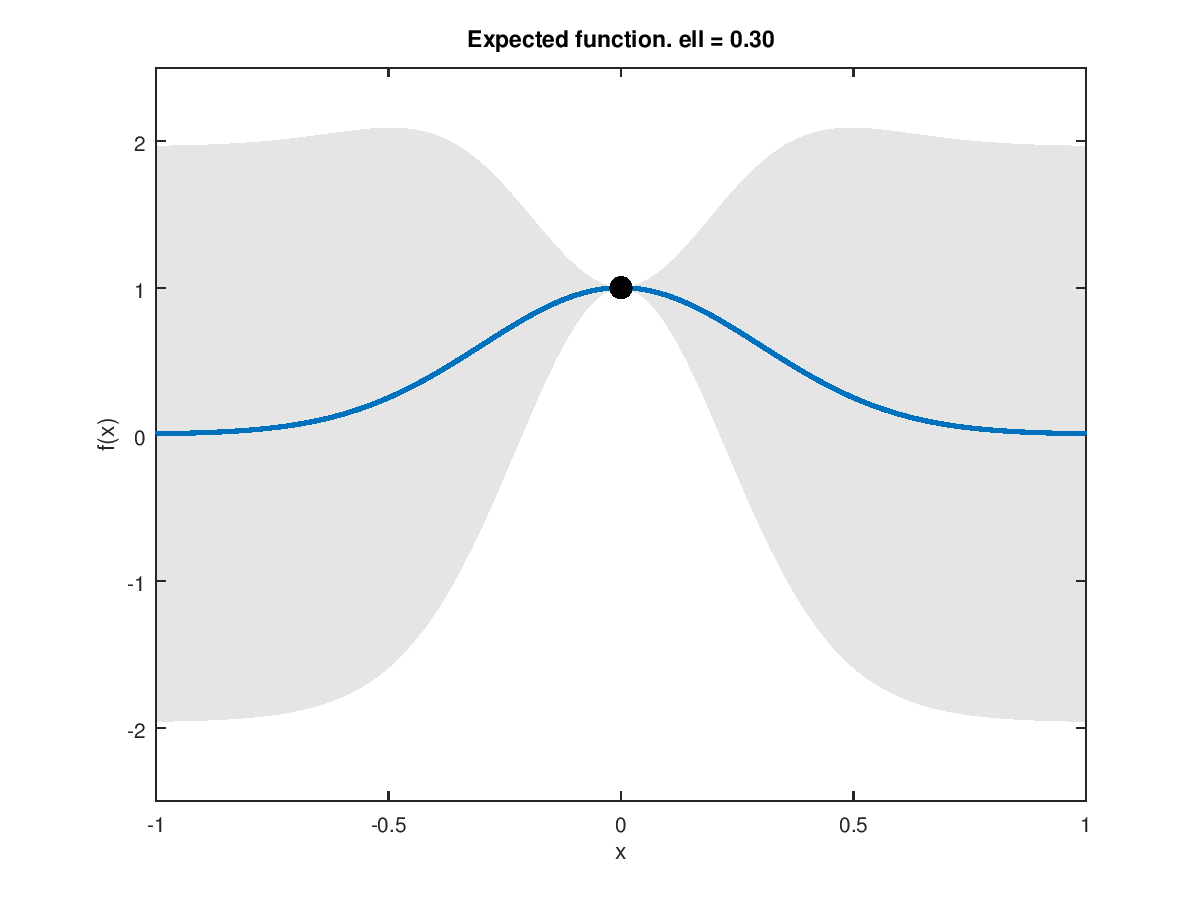The gray area gives us a measure of the certainty of the expected function meaning that the function could be just about anything outside the measurement point, something which can be seen in the examples where we draw functions from the distribution above.

When we have three measurements present the expected function is reasonably sure between the points, but the uncertainty quickly increases where there is no data present. The expected function converges to the mean function at the edges (which is zero in this case).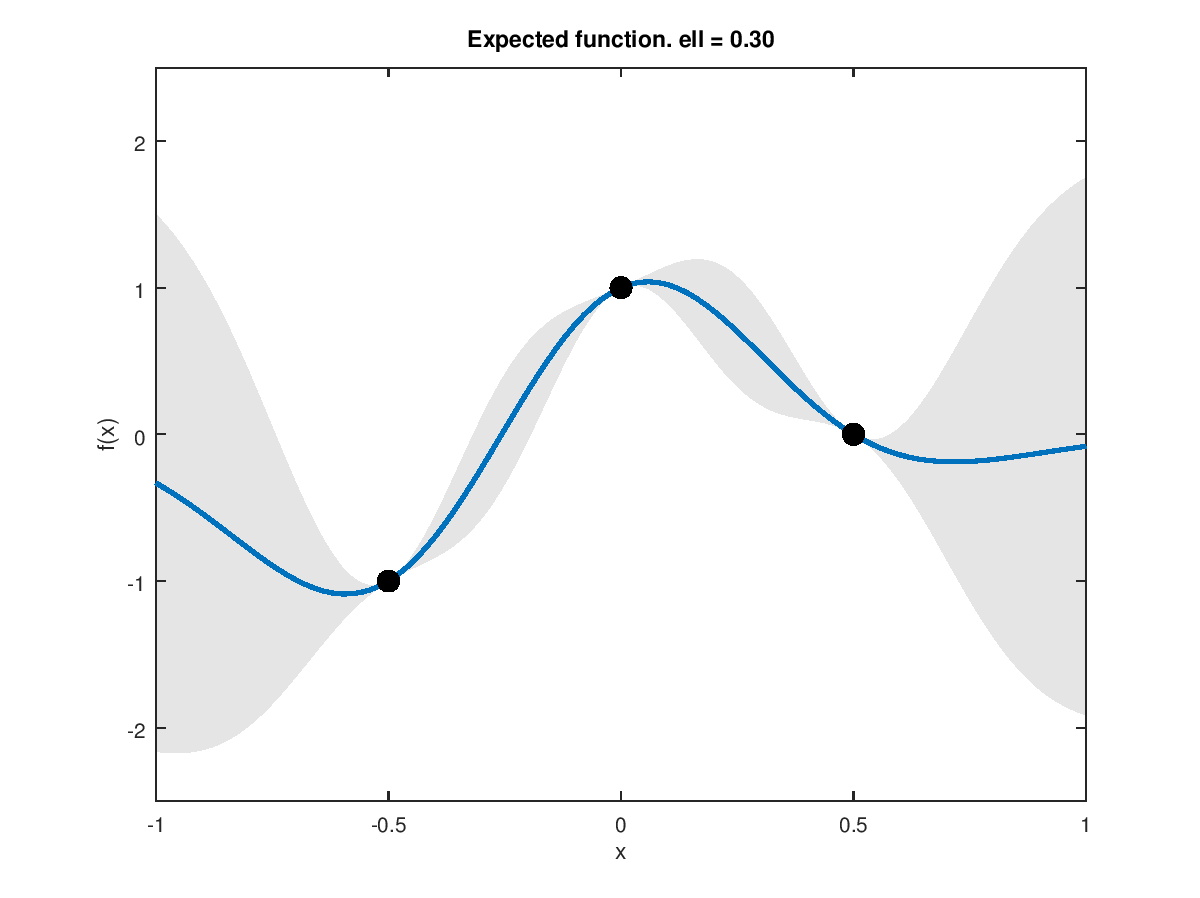For computer experiments the measurements are exact, but for real world measurements, we can seldom exclude noise from the equation.

## Kernel Hyperparameters

We can add some slack to the regression by writing the kernel function as$k_yleft(x_p, x_qright) = sigma^2_f exp left(-frac{1}{2ell^2}left(x_p - x_qright)^2right) + sigma^2_n delta_{pq}$

where$ell$ is the length-scale,$sigma^2_f$ is the signal variance and$sigma^2_n$ is the variance of the noise. These three parameters are called “hyperparameters” and are used to find the best fit of the expected function with respect to some measure.

The figures below show what happens when the three parameters are varied. The function variance is the prior variance when no measurements have been obtained and are changed for each column; the noise variance is changed row-wise and the length-scale changes per image.

This slideshow requires JavaScript.

## Gaussian Processes in Practice

Gaussian Processes works well for many dimensions of data. The drawback is expensive calculations involving a matrix inversion of the part of the covariance where the measurement data is located. If we have many measurements, it quickly becomes computationally intractable to perform the inversion concerning both computational complexity and computer memory requirements.

The inversion has to be performed many times since the hyperparameters are optimized in order to obtain the best fit for the regression. The optimization process is in general nonlinear since the log marginal likelihood is used.

One way to go around this problem is to truncate the covariance function to zero for small values and use sparse matrices and solving calculations involving matrix inversions using conjugate gradient methods. There are other ways to approximate the problem as well.

We will describe other aspects of Gaussian Processes in future postings.

This site uses Akismet to reduce spam. Learn how your comment data is processed.